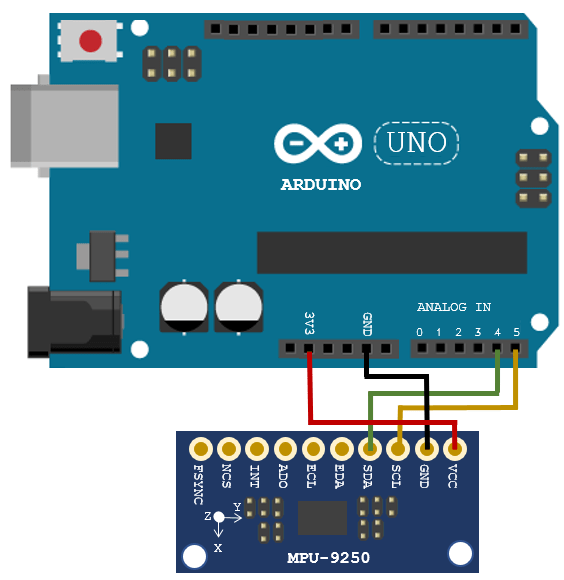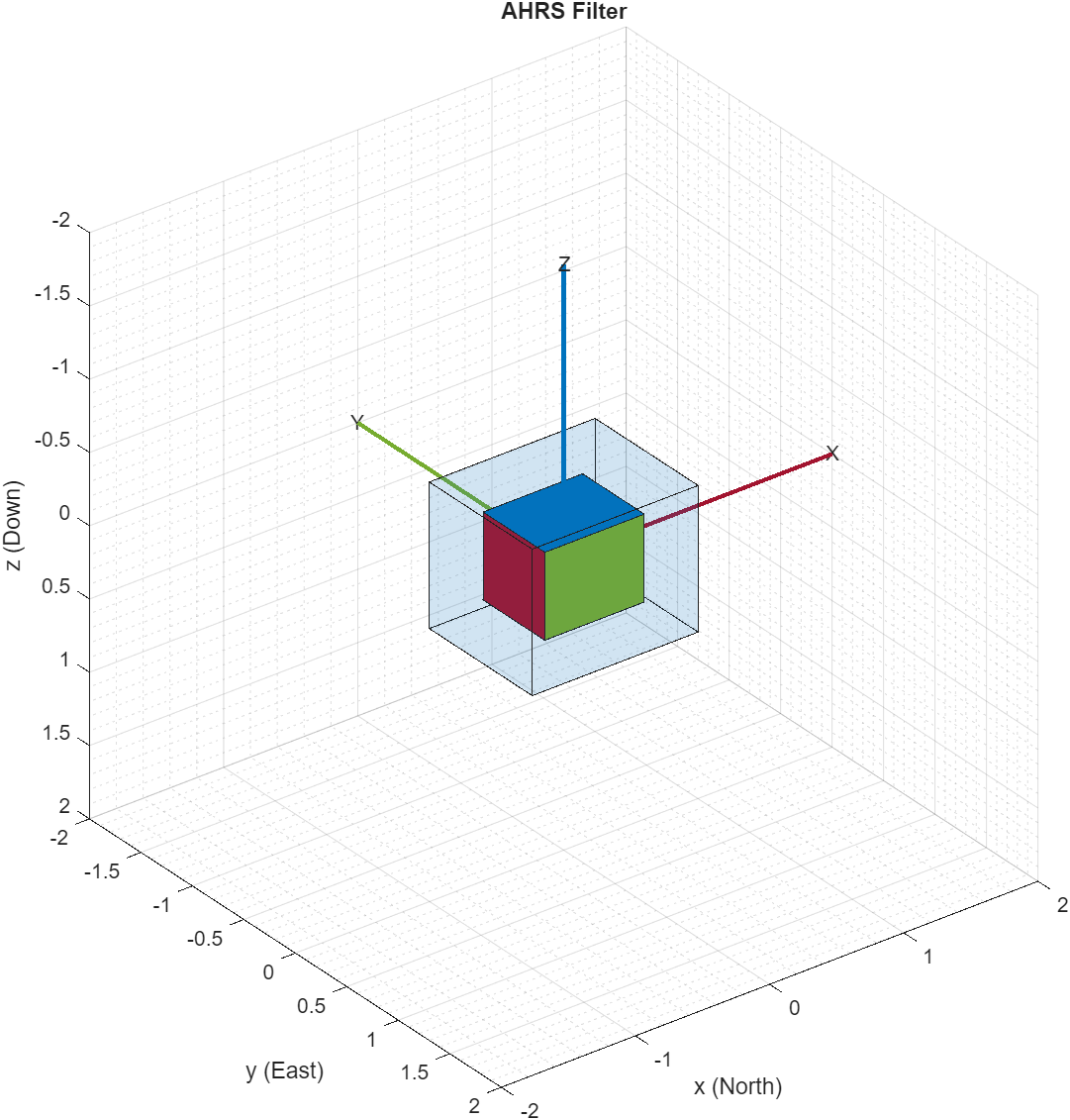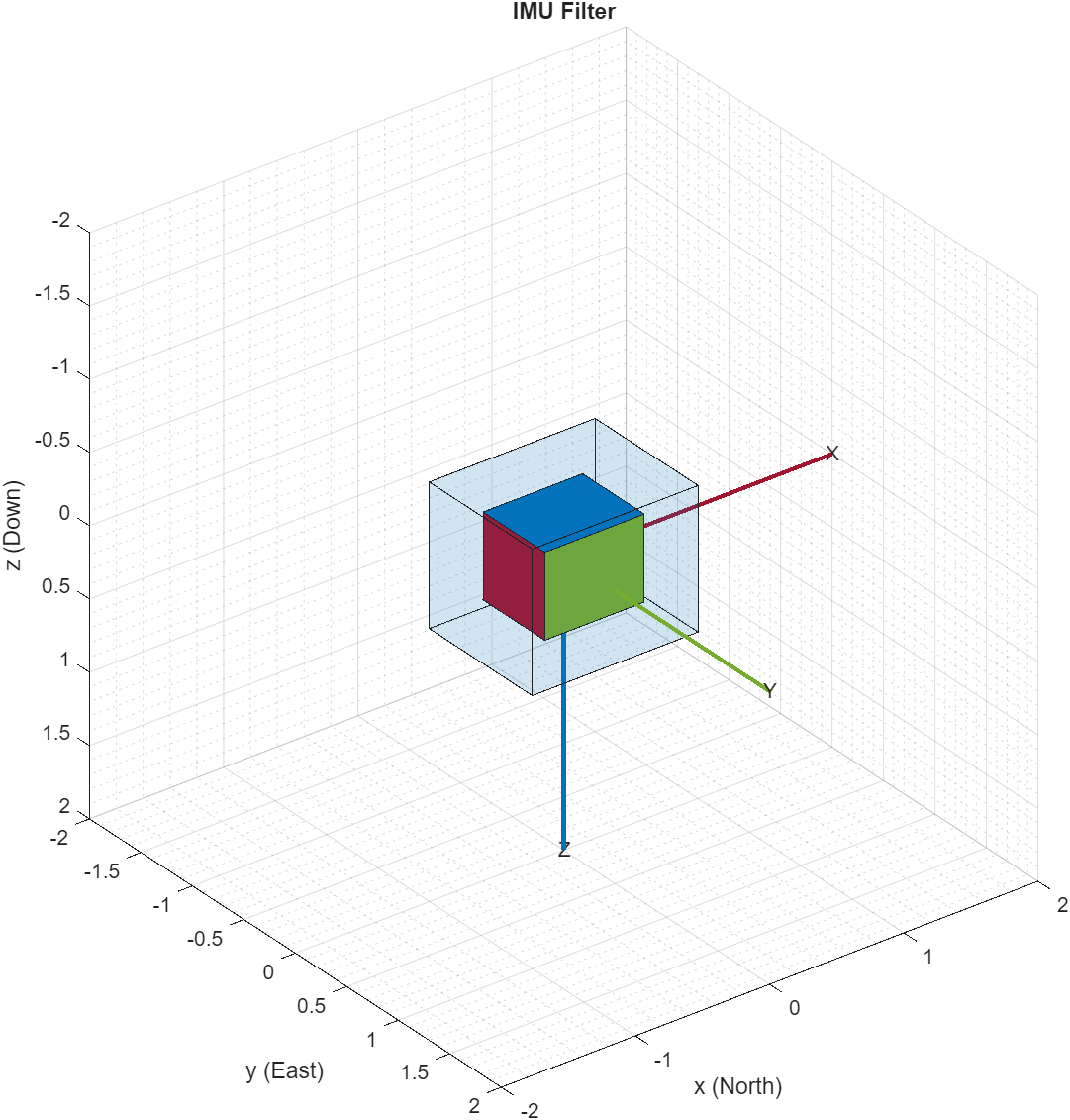# Estimating Orientation Using Inertial Sensor Fusion and MPU-9250

This example shows how to get data from an InvenSense MPU-9250 IMU sensor, and to use the 6-axis and 9-axis fusion algorithms in the sensor data to compute orientation of the device.

MPU-9250 is a 9-axis sensor with accelerometer, gyroscope, and magnetometer. The accelerometer measures acceleration, the gyroscope measures angular velocity, and the magnetometer measures magnetic field in x-, y- and z- axis. The axis of the sensor depends on the make of the sensor.

### Required MathWorks® Products

• MATLAB®

• MATLAB Support Package for Arduino® Hardware

• Navigation Toolbox™ or Sensor Fusion and Tracking Toolbox™

### Required Hardware

• Arduino Uno

• InvenSense MPU-9250

### Hardware Connection

Connect the SDA, SCL, GND, and the VCC pins of the MPU-9250 sensor to the corresponding pins on the Arduino® Hardware. This example uses the Arduino Uno board with the following connections:

• SDA - A4

• SCL - A5

• VCC - +3.3V

• GND - GNDEnsure that the connections to the sensors are intact. It is recommended to use a prototype shield and solder the sensor to it to avoid loose connections while moving the sensor. Refer the Troubleshooting Sensors (MATLAB Support Package for Arduino Hardware) page for sensors to debug the sensor related issues.

### Create Sensor Object

Create an arduino object and include the I2C library.

a = arduino('COM9', 'Uno', 'Libraries', 'I2C');
Updating server code on board Uno (COM9). This may take a few minutes. 

Create the MPU-9250 sensor object.

fs = 100; % Sample Rate in Hz imu = mpu9250(a,'SampleRate',fs,'OutputFormat','matrix'); 

### Compensating Magnetometer Distortions

Fusion algorithms use magnetometer readings which need to be compensated for magnetic distortions. In general, two effects exist: Hard iron distortions and soft iron distortions. To learn more about this distortion, refer to More About (Navigation Toolbox). These distortions can be corrected by using the correction values that can be determined using these steps:

1. Rotate the sensor from 0 to 360 degree along each axis.

2. Use the magcal (Navigation Toolbox) function, as shown below, to obtain the correction coefficients.

ts = tic; stopTimer = 50; magReadings=[]; while(toc(ts) < stopTimer) % Rotate the sensor along x axis from 0 to 360 degree. % Take 2-3 rotations to improve accuracy. % For other axes, rotate along that axes. [accel,gyro,mag] = read(imu); magReadings = [magReadings;mag]; end [A, b] = magcal(magReadings); % A = 3x3 matrix for soft iron correction % b = 3x1 vector for hard iron correction 

### Aligning the axis of MPU-9250 sensor with NED Coordinates

Sensor fusion algorithms used in this example use North-East-Down(NED) as a fixed, parent coordinate system. In the NED reference frame, the X-axis points north, the Y-axis points east, and the Z-axis points down. Depending on the algorithm, north may either be the magnetic north or true north. The algorithms in this example use the magnetic north. The algorithms used here expects all the sensors in the object to have their axis aligned and is in accordance with NED convention.

MPU-9250 has two devices, the magnetometer and the accelerometer-gyroscope, on the same board. The axes of these devices are different from each other. The magnetometer axis is aligned with the NED coordinates. The axis of the accelerometer-gyroscope is different from magnetometer in MPU-9250. The accelerometer and the gyroscope axis need to be swapped and/or inverted to match the magnetometer axis. For more information refer to the section “Orientation of Axes” section in MPU-9250 datasheet.To align MPU-9250 accelerometer-gyroscope axes to NED coordinates, do the following:

1. Define device axes: Define the imaginary axis as the device axis on the sensor in accordance to NED coordinate system which may or may not be same as sensor axes. For MPU-9250, magnetometer axis can be considered as device axis.

2. Swap the x and y values of accelerometer and gyroscope readings, so that the accelerometer and gyroscope axis is aligned with magnetometer axis.

3. Determine polarity values for accelerometer, and gyroscope.

a. Accelerometer

• Place the sensor such that device X axis is pointing downwards, perpendicular to the surface at which sensor is kept. Accelerometer readings should read approximately [9.8 0 0]. If not negate the x-values of accelerometer.

• Place the sensor such that device Y axis is pointing downwards, perpendicular to the surface at which sensor is kept. Accelerometer readings should read approximately [0 9.8 0]. If not negate the y-values of accelerometer.

• Place the sensor such that device Z axis is pointing downwards, perpendicular to the surface at which sensor is kept. Accelerometer readings should read approximately [0 0 9.8]. If not negate the z-values of accelerometer.

b. Gyroscope

Rotate the sensor along each axis and capture the readings. Use the right hand screw rule to correct the polarity of rotation.

The above method is used to set the axis of the sensor in this example.

### Tuning Filter Parameters

The algorithms used in this example, when properly tuned, enable estimation of orientation and are robust against environmental noise sources. You must consider the situations in which the sensors are used and tune the filters accordingly. See Custom Tuning of Fusion Filters for more details related to tuning filter parameters.

The example demonstrates three algorithms to determine orientation, namely ahrsfilter, imufilter, and ecompass. Refer Determine Orientation Using Inertial Sensors for more details related to inertial fusion algorithms.

### Accelerometer-Gyroscope-Magnetometer Fusion

An attitude and heading reference system (AHRS) consist of a 9-axis system that uses an accelerometer, gyroscope, and magnetometer to compute orientation of the device. The ahrsfilter produces a smoothly changing estimate of orientation of the device, while correctly estimating the north direction. The ahrsfilter has the ability to remove gyroscope bias and can also detect and reject mild magnetic jamming.

The following code snippets use ahrsfilter system object to determine orientation of the sensor and creates a figure which gets updated as you move the sensor. The sensor has to be stationary, before the start of this example.

% GyroscopeNoise and AccelerometerNoise is determined from datasheet. GyroscopeNoiseMPU9250 = 3.0462e-06; % GyroscopeNoise (variance value) in units of rad/s AccelerometerNoiseMPU9250 = 0.0061; % AccelerometerNoise(variance value)in units of m/s^2 viewer = HelperOrientationViewer('Title',{'AHRS Filter'}); FUSE = ahrsfilter('SampleRate',imu.SampleRate, 'GyroscopeNoise',GyroscopeNoiseMPU9250,'AccelerometerNoise',AccelerometerNoiseMPU9250); stopTimer = 100;

While the below code is getting executed, slowly move the sensor and check if the motion in the figure matches the motion of the sensor.

% The correction factors A and b are obtained using magcal function as explained in one of the % previous sections, 'Compensating Magnetometer Distortions'. magx_correction = b(1); magy_correction = b(2); magz_correction = b(3); ts = tic; while(toc(ts) < stopTimer) [accel,gyro,mag] = read(imu); % Align coordinates in accordance with NED convention accel = [-accel(:,2), -accel(:,1), accel(:,3)]; gyro = [gyro(:,2), gyro(:,1), -gyro(:,3)]; mag = [mag(:,1)-magx_correction, mag(:, 2)- magy_correction, mag(:,3)-magz_correction] * A; rotators = FUSE(accel,gyro,mag); for j = numel(rotators) viewer(rotators(j)); end end

When the device X axis of sensor is pointing to north, the device Y-axis is pointing to east and device Z-axis is pointing down.When the device X axis of sensor is pointing to north, device Y-axis is pointing to west and device Z-axis is pointing upwards.### Accelerometer-Gyroscope Fusion

The imufilter system object fuses accelerometer and gyroscope data using an internal error-state Kalman filter. The filter is capable of removing the gyroscope bias noise, which drifts over time. The filter does not process magnetometer data, so it does not correctly estimate the direction of north. The algorithm assumes the initial position of the sensor is in such a way that device X-axis of the sensor is pointing towards magnetic north, the device Y-axis of the sensor is pointing to east and the device Z-axis of the sensor is pointing downwards. The sensor must be stationary, before the start of this example.

The following code snippets use imufilter object to determine orientation of the sensor and creates a figure which gets updated as you move the sensor.

displayMessage(['This section uses IMU filter to determine orientation of the sensor by collecting live sensor data from the \slmpu9250 \rm' ... 'system object. Move the sensor to visualize orientation of the sensor in the figure window. Keep the sensor stationery before you'... 'click OK'],... 'Estimate Orientation using IMU filter and MPU-9250.') % GyroscopeNoise and AccelerometerNoise is determined from datasheet. GyroscopeNoiseMPU9250 = 3.0462e-06; % GyroscopeNoise (variance) in units of rad/s AccelerometerNoiseMPU9250 = 0.0061; % AccelerometerNoise (variance) in units of m/s^2 viewer = HelperOrientationViewer('Title',{'IMU Filter'}); FUSE = imufilter('SampleRate',imu.SampleRate, 'GyroscopeNoise',GyroscopeNoiseMPU9250,'AccelerometerNoise', AccelerometerNoiseMPU9250); stopTimer=100;

While the below code is getting executed, slowly move the sensor and check if the motion in the figure matches the motion of the sensor.

% Use imufilter to estimate orientation and update the viewer as the sensor moves for time specified by stopTimer tic; while(toc < stopTimer) [accel,gyro] = read(imu); accel = [-accel(:,2), -accel(:,1), accel(:,3)]; gyro = [gyro(:,2), gyro(:,1), -gyro(:,3)]; rotators = FUSE(accel,gyro); for j = numel(rotators) viewer(rotators(j)); end end 

The imufilter algorithm can also be used with MPU6050 as well, since it does not require magnetometer values.

When the device X axis of sensor is pointing to north, device Z-axis is pointing downwards and device Y-axis is pointing to east.When the device X axis of sensor is pointing upwards, device Y-axis is points to west and device Z-axis points to south.### Accelerometer-Magnetometer Fusion

The ecompass system object fuses the accelerometer and magnetometer data. The Ecompass algorithm is a memoryless algorithm that requires no parameter tuning but is highly susceptible to sensor noise. You could use spherical linear interpolation (SLERP) to lowpass filter a noisy trajectory. Refer Lowpass Filter Orientation Using Quaternion SLERP example for more details.

displayMessage(['This section uses \slecompass \rmfunction to determine orientation of the sensor by collecting live sensor data from the \slmpu9250 ' ... '\rmsystem object. Move the sensor to visualize orientation of the sensor in the figure window. Keep the sensor stationery before you click OK'],... 'Estimate Orientation using Ecompass algorithm.') viewer = HelperOrientationViewer('Title',{'Ecompass Algorithm'}); % Use ecompass algorithm to estimate orientation and update the viewer as the sensor moves for time specified by stopTimer. % The correction factors A and b are obtained using magcal function as explained in one of the % previous sections, 'Compensating Magnetometer Distortions'. magx_correction = b(1); magy_correction = b(2); magz_correction = b(3); stopTimer = 100; tic; while(toc < stopTimer) [accel,~,mag] = read(imu); accel = [-accel(:,2), -accel(:,1), accel(:,3)]; mag = [mag(:,1)-magx_correction, mag(:, 2)- magy_correction, mag(:,3)-magz_correction] * A; rotators = ecompass(accel,mag); for j = numel(rotators) viewer(rotators(j)); end end 

### Clean Up

When the connection is no longer needed, release and clear the objects

release(imu); clear;

### Things to try

You can try this example with other sensors such as InvenSense MPU-6050 and STMicroelectronics LSM9DS1. Note that the MPU-6050 sensor can be used only with the imufilter system object.